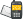It is currently 21 Mar 2019, 11:41### GMAT Club Daily Prep

#### Thank you for using the timer - this advanced tool can estimate your performance and suggest more practice questions. We have subscribed you to Daily Prep Questions via email.

Customized
for You

we will pick new questions that match your level based on your Timer History

Track

every week, we’ll send you an estimated GMAT score based on your performance

Practice
Pays

we will pick new questions that match your level based on your Timer History

#### Not interested in getting valuable practice questions and articles delivered to your email? No problem, unsubscribe here.# abc > 0Question banks Downloads My Bookmarks Reviews Important topics
Author Message
TAGS:
ModeratorJoined: 18 Apr 2015
Posts: 5856
Followers: 94

Kudos [?]: 1145 , given: 5455

abc > 0 [#permalink]
Expert's post00:00

Question Stats:88% (00:40) correct12% (00:07) wrongbased on 25 sessions
$$abc > 0$$

 Quantity A Quantity B c(a + b) abc

A) Quantity A is greater.
B) Quantity B is greater.
C) The two quantities are equal.
D) The relationship cannot be determined from the information given.
[Reveal] Spoiler: OA

_________________InternJoined: 07 Jan 2019
Posts: 31
Followers: 0

Kudos [?]: 17  , given: 9

Re: abc > 0 [#permalink]
1
KUDOS
Given:
abc > 0 which implies that,
Out of a,b,c either two of the variables can be negative or all of the three variables can be positive.
Suppose a=1, b=2 and c=3.
We get, A > B ( A=9 and B=6)
Suppose a= -1, b=-2 and c=3
We get, B > A ( A=-9 and B=3)
Hence, the answer is D.
Please rectify if the process is wrong.

Posted from my mobile deviceSupreme Moderator
Joined: 01 Nov 2017
Posts: 370
Followers: 5

Kudos [?]: 107 , given: 4

Re: abc > 0 [#permalink]
Expert's post
Carcass wrote:
$$abc > 0$$

 Quantity A Quantity B c(a + b) abc

A) Quantity A is greater.
B) Quantity B is greater.
C) The two quantities are equal.
D) The relationship cannot be determined from the information given.

A very straight forward solution, even if we take all as positive..
(1) Let $$a=b=c=3$$
A=(c(a + b))=$$3(3+3)=18$$
B=(abc)=$$3*3*3=27$$
so B>A
(2) Let $$a=b=c=1$$
A=(c(a + b))=$$1(1+1)=2$$
B=(abc)=$$1*1*1=1$$
so A>B

D
_________________

Some useful Theory.
1. Arithmetic and Geometric progressions : https://greprepclub.com/forum/progressions-arithmetic-geometric-and-harmonic-11574.html#p27048
2. Effect of Arithmetic Operations on fraction : https://greprepclub.com/forum/effects-of-arithmetic-operations-on-fractions-11573.html?sid=d570445335a783891cd4d48a17db9825
3. Remainders : https://greprepclub.com/forum/remainders-what-you-should-know-11524.html
4. Number properties : https://greprepclub.com/forum/number-property-all-you-require-11518.html
5. Absolute Modulus and Inequalities : https://greprepclub.com/forum/absolute-modulus-a-better-understanding-11281.htmlRe: abc > 0   [#permalink] 12 Jan 2019, 04:36
Display posts from previous: Sort by

# abc > 0Question banks Downloads My Bookmarks Reviews Important topicsPowered by phpBB © phpBB Group Kindly note that the GRE® test is a registered trademark of the Educational Testing Service®, and this site has neither been reviewed nor endorsed by ETS®.